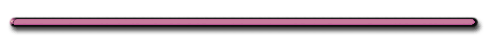HomeOptical Illusion

Is the Acceleration
Constant, Increasing or Zero?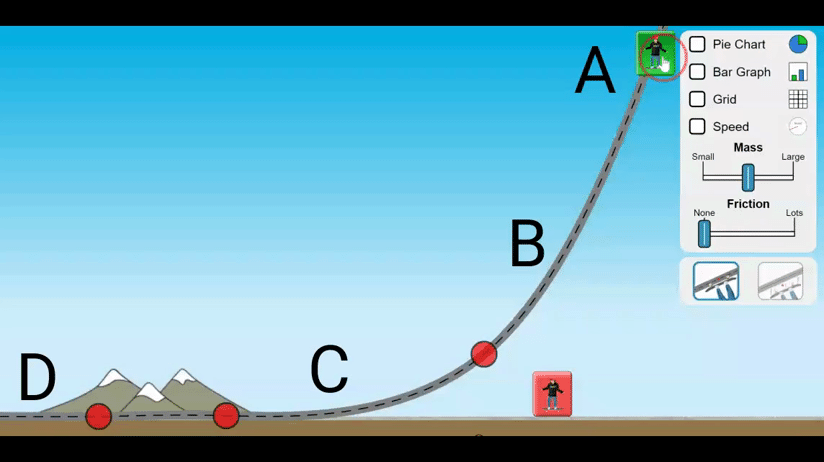A - B, B - C, C - D

Video Instruction

showmethephysics.com

 Ranking Task Rank the acceleration from greatest to least. Ties are possible. Explain 0 - 3____ 3 - 6____ 6 - 8____ 8 - 9____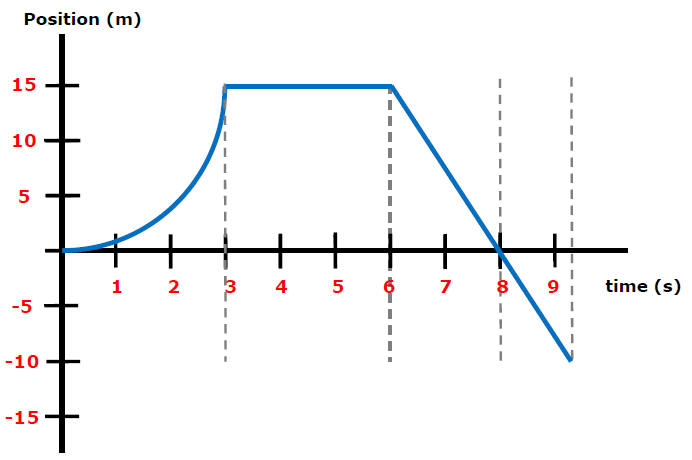Describe the Acceleration BelowReview

Describe the motion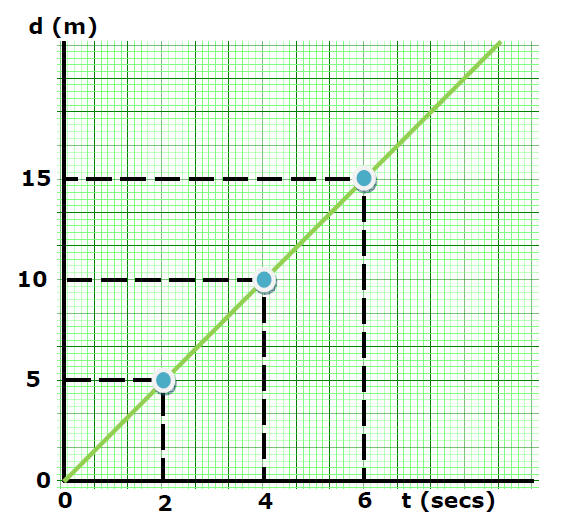Slope: =

d/t = vel.

velocity is constant +

3.  a = 0 (vel. not changing)

Ex)

 d (cm)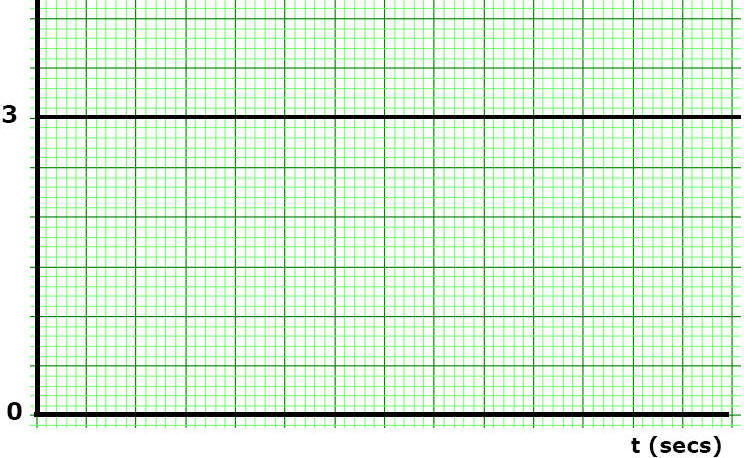1. y changes:

2. Slope: =

1. Y changes:

d constant

2. Slope:

d/t = v

v = 0

Ex)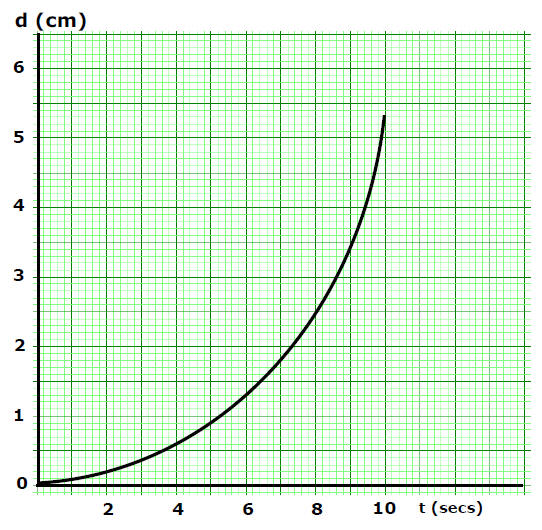1. y changes:

2. Slope: =

1. Y changes: d inc.

2. Slope: d/t = v

V is increasing

3. Constant accel.

(smooth increase of v)

Ex)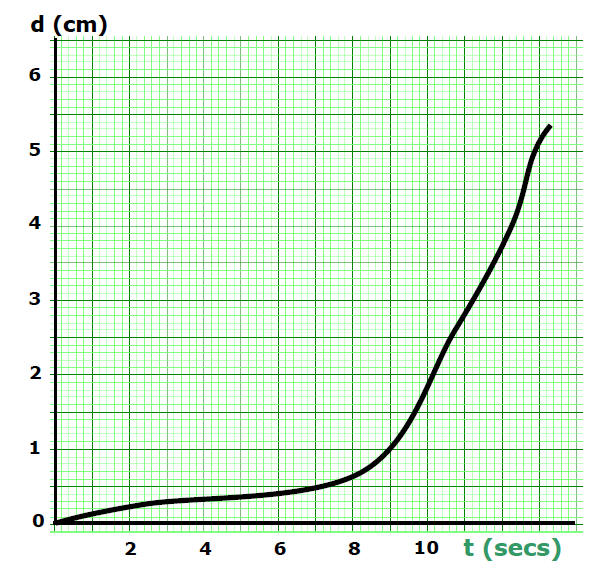1. y changes:

2. Slope: =

1. Y changes: d inc.

2. Slope: d/t = v

V is increasing

3. Not Constant acceleration

Ex)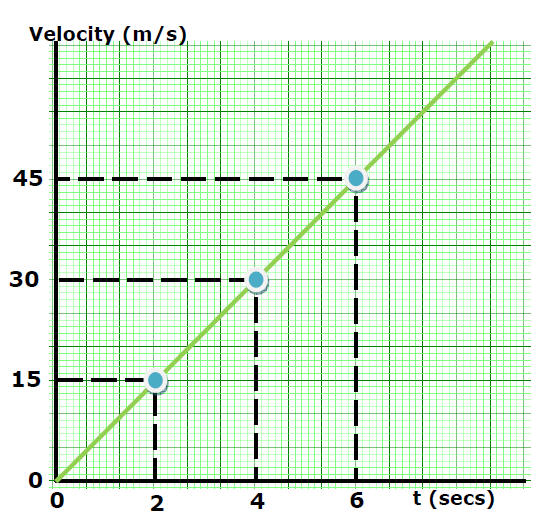1. y changes:

2. Slope: =

1. y changes:

increasing v

2. Slope:

v/t = a

acceleration is constant +Ex)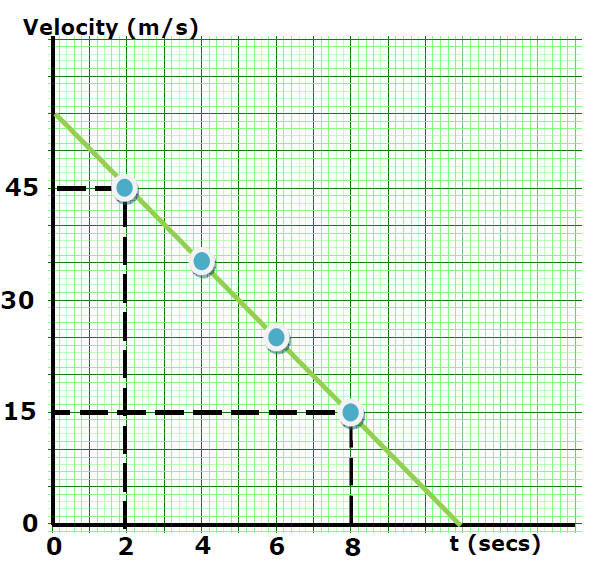1. y changes:

2. Slope: =

1. y changes: decreasing v

2. Slope: v/t = a

acceleration is constant and neg.

 Try this great Kinematics graphs game(3) 10 m/s2***AP Extra Plots**